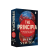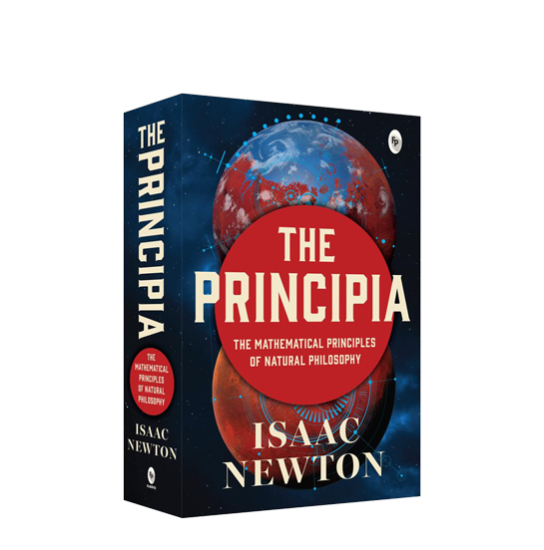•Isaac Newton

A groundbreaking work in the field of physics and mathematics. In this monumental work, Newton formulated the laws of motion and universal gravitation, laying the foundation for classical mechanics a

A groundbreaking work in the field of physics and mathematics. In this monumental work, Newton formulated the laws of motion and universal gravitation, laying the foundation for classical mechanics and revolutionizing our understanding of the physical world. The Principia remains one of the most significant scientific books ever written, influencing generations of scientists, and shaping the course of modern physics and mathematics. The Groundbreaking Work of Sir Isaac Newton • Mathematical proofs and equations. • Comprehensive coverage of planetary motion. • Helps in understanding the principles of motion. • Logical and rigorous approach to scientific inquiry. • Studied and revered as a seminal work in the field of science.Sir Isaac Newton (1643–1727), an English mathematician, physicist, and astronomer, is a towering figure in the history of science. Knighted by Queen Anne, Newton's profound contributions continue to shape scientific inquiry and inspire subsequent generations. His genius and relentless curiosity continue to influence a wide array of disciplines, leaving an enduring impact on human knowledge.

ISBN-13

9789358562163

12+

Language

English

Item Weight(gm)

503

Dimensions(cm)

12.7 x 19.6 x 4.5

Paperback

716

From the Publisher